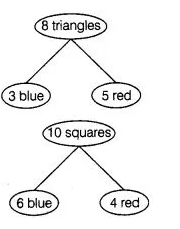# A child’s game has 8 triangles of

Question:

A child’s game has 8 triangles of which 3 are blue and rest are red, and 10 squares of which 6 are blue and rest are red. One piece is lost at random.

Find the probability that it is a

(i) triangle

(ii) square

(iii)square of blue colour

(iv) triangle of red colour

Solution:

Total number of figures

$n(S)=8$ triangles $+10$ squares $=18$(i) $P$ (lost piece is a triangle) $=\frac{8}{18}=\frac{4}{9}$

(ii) $P$ (lost piece is a square) $=\frac{10}{18}=\frac{5}{9}$

(iii) $P$ (square of blue colour) $=\frac{6}{18}=\frac{1}{3}$

(iv) $P$ (triangle of red colour) $=\frac{5}{18}$